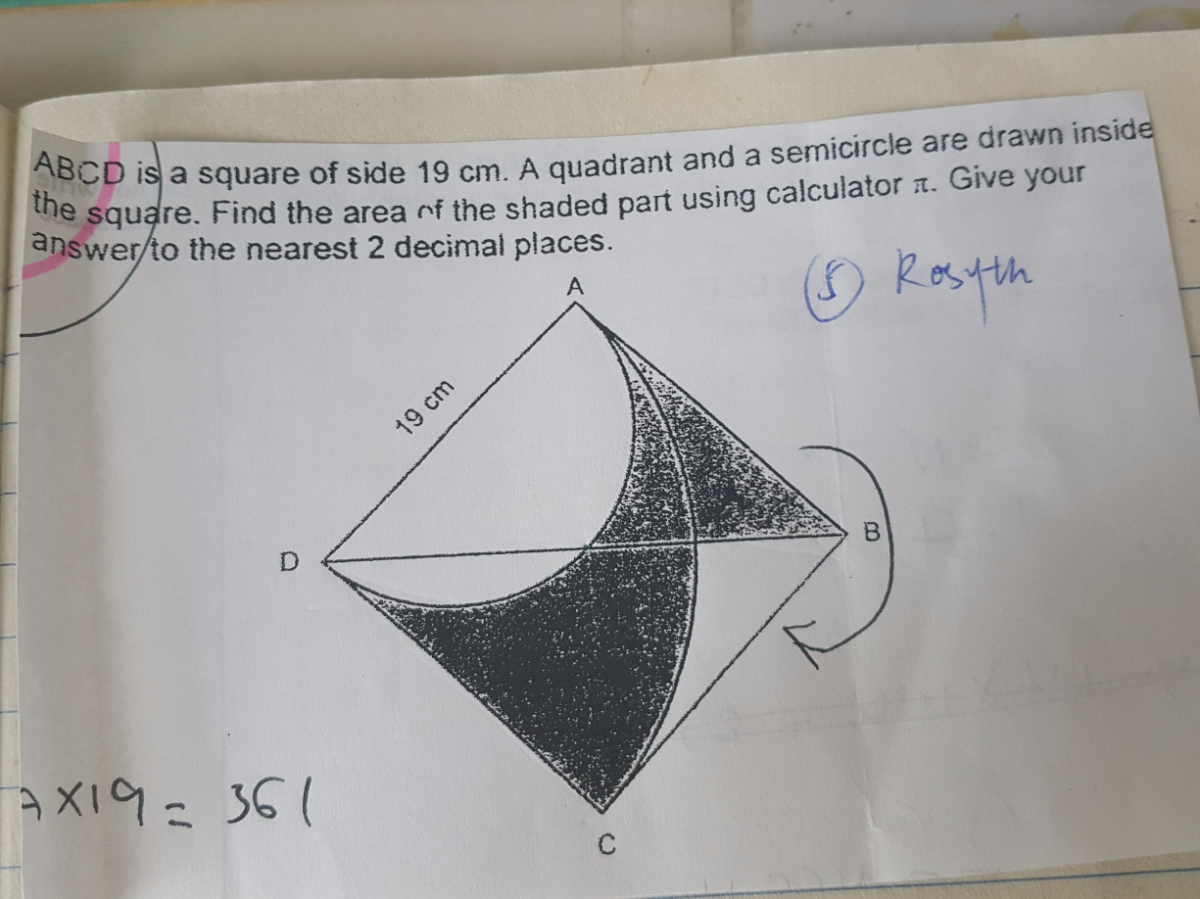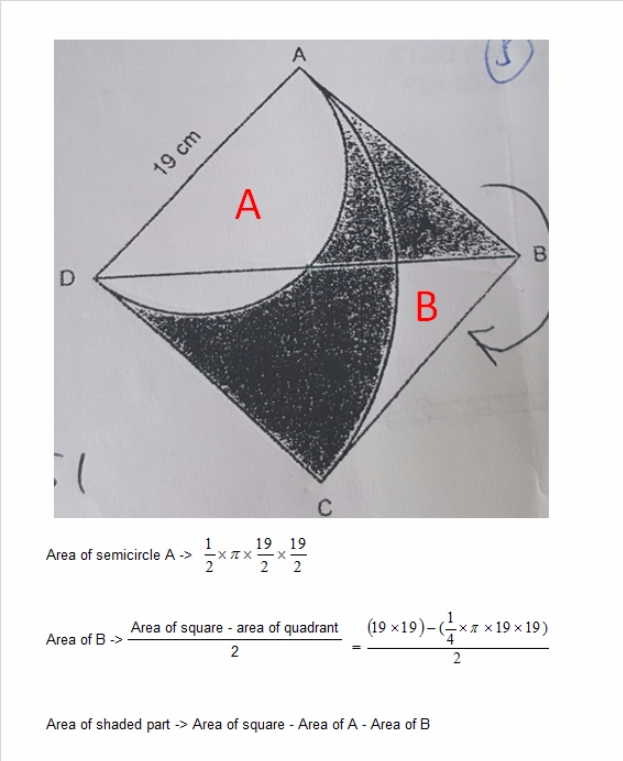# QuestionSource: RosythVery appreciate

0 Replies 0 Likes

I would solve the question as follows :

area of square ——- 19 x 19 = 361
area of quadrant ——- (1/4) x pi x 19 x 19 = 90.25pi
area of semi circle ——- (1/2) x pi x (19/2) x 19/2) = 45.125pi
area of shaded part ——- 90.25pi – 45.125pi + (361 – 90.25pi)/2 = 180.50

Ans : 180.50 sq cm.

0 Replies 0 Likes